Home » Education » Statistics Assignment Example

# Statistics Assignment Example

74 views

Group Project – Math

 127.12 147.58 143.66 134.05 182.58 107.6 145.4 45.03 151.81 134.95 143.93 129.82 197.16 114.45 140.28 126.03 210.45 88.12 120.67 161.86 71.13 144.42 132.16 121.48 103.5 116.56 69.54 34.75 106.48 208.67 138.45 84.64 126.92 118.71 148.33 181.5 78.26 127.23 202.38 180.2 132.99 127.12 147.58 143.66 134.05 182.58 107.6 145.4 45.03 151.81

The following​​ table represents the summary statistics for​​ the above data, and it can be seen in the table that the total number of values is 50, while there is no missing​​ value in the data. The mean value is 129.9368, which is greater than the mean value of the previous​​ downloading rate of​​ 115 Mbps.​​ The minimum amount in the data is 34.5, while the maximum amount is​​ 210.45. The​​ Sum of all values is 6496.84.​​ The standard error of mean and standard error of deviation is​​ 5.57571 and 39.42623, respectively.​​ The rest of the statistics can be observed in the following table:

Table 2: Summary Statistics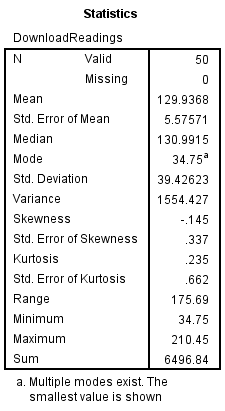Moreover, the following table represents the​​ frequency, percent, good percent, and cumulative percent.

Table 3:​​ Frequency and Percentage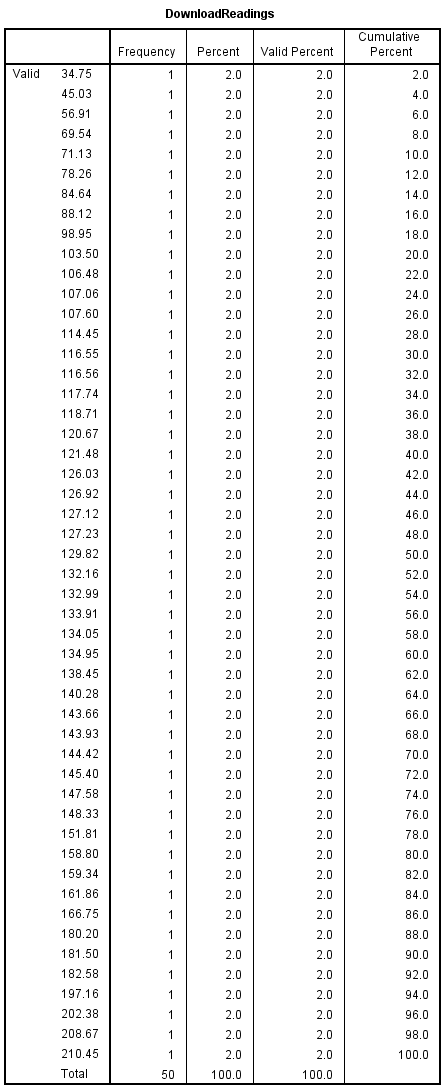The graphical representation of the statistical description can be observed in the following histogram figure:

Figure 1:​​ Graphical Summary Statistics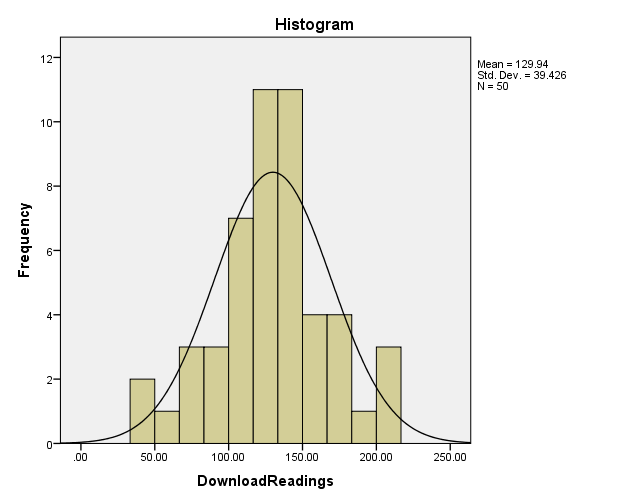• Using the 95%​​ level of significance,​​ the following hypothesis is conducted to determine whether the claims of manufacture are valid or not:

H0​​ : µ = 0

H1: µ​​ ​​ 0

The following two tables show the result of​​ one sample t-test:

Table 4: One-sample Statistics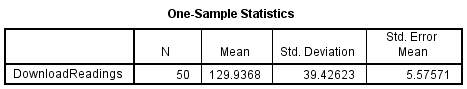Table 5: One-Sample Test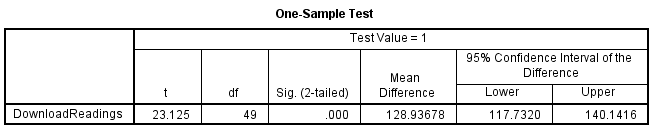Moving from left to right,​​ the data is given​​ with the observed t-value, i.e.,​​ 23.125,​​ the degree​​ of freedom, i.e., 49, and the p-value or statistical​​ significance. We can see that the value of p is the value of is the value of is less than 0.05, i.e.,​​ 0.000. Hence, it is concluded that​​ the claims of manufacture​​ are valid as the null hypothesis cannot be rejected in favor of the alternate view.​​ The downloading rates of the new connection will be improved.

• Recommendations

It is suggested, based on the​​ results, to adopt the new connection as the measured​​ mean​​ download rate​​ of a new relationship​​ from various​​ locations on the company website is better than the​​ previous link's mean download rate. Furthermore, the hypothesis test has proved that the​​ manufacturer's claims are valid that the download rates will be improved.​​ So the company should move on with the new internet connection.